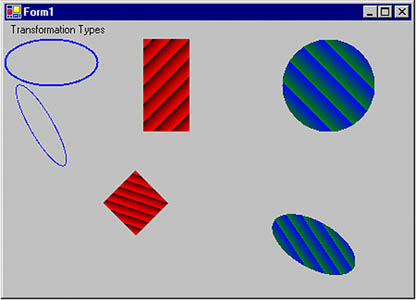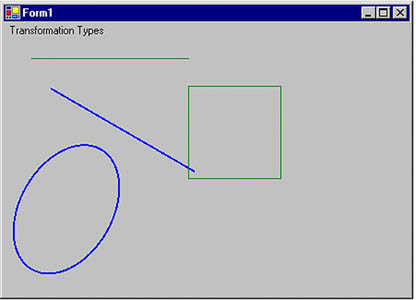# Global, Local, and Composite Transformations

Transformations can be divided into two categories based on their scope: global and local. In addition, there are composite transformations. A global transformation is applicable to all items of a Graphics object. The Transform property of the Graphics class is used to set global transformations.

A composite transformation is a sequence of transformations. For example, scaling followed by translation and rotation is a composite translation. The MultiplyTransform, RotateTransform, ScaleTransform, and TranslateTransform methods are used to generate composite transformations.

Listing 10.14 draws two ellipses and a rectangle, then calls ScaleTransform, TranslateTransform, and RotateTransform (a composite transformation). The items are drawn again after the composite transformation.

Listing 10.14 Applying a composite transformation

```private void GlobalTransformation_Click(object sender,
System.EventArgs e)
{
// Create a Graphics object
Graphics g = this.CreateGraphics();
g.Clear(this.BackColor);
// Create a blue pen with width of 2
Pen bluePen = new Pen(Color.Blue, 2);
Point pt1 = new Point(10, 10);
Point pt2 = new Point(20, 20);
Color [] lnColors = {Color.Black, Color.Red};
Rectangle rect1 = new Rectangle(10, 10, 15, 15);
// Create two linear gradient brushes
(rect1, Color.Blue, Color.Green,
(pt1, pt2, Color.Red, Color.Green);
// Set linear colors
lgBrush.LinearColors = lnColors;
// Set gamma correction
lgBrush.GammaCorrection = true;
// Fill and draw rectangle and ellipses
g.FillRectangle(lgBrush, 150, 0, 50, 100);
g.DrawEllipse(bluePen, 0, 0, 100, 50);
g.FillEllipse(lgBrush1, 300, 0, 100, 100);
// Apply scale transformation
g.ScaleTransform(1, 0.5f);
// Apply translate transformation
g.TranslateTransform(50, 0, MatrixOrder.Append);
// Apply rotate transformation
g.RotateTransform(30.0f, MatrixOrder.Append);
// Fill ellipse
g.FillEllipse(lgBrush1, 300, 0, 100, 100);
// Rotate again
g.RotateTransform(15.0f, MatrixOrder.Append);
// Fill rectangle
g.FillRectangle(lgBrush, 150, 0, 50, 100);
// Rotate again
g.RotateTransform(15.0f, MatrixOrder.Append);
// Draw ellipse
g.DrawEllipse(bluePen, 0, 0, 100, 50);
// Dispose of objects
lgBrush1.Dispose();
lgBrush.Dispose();
bluePen.Dispose();
g.Dispose();
}
```

Figure 10.15 shows the output from Listing 10.14.

Figure 10.15. Composite transformationA local transformation is applicable to only a specific item of a Graphics object. The best example of local transformation is transforming a graphics path. The Translate method of the GraphicsPath class translates only the items of a graphics path. Listing 10.15 translates a graphics path. We create a Matrix object and apply rotate and translate transformations to it.

Listing 10.15 Translating graphics path items

```private void LocalTransformation_Click(object sender,
System.EventArgs e)
{
// Create a Graphics object
Graphics g = this.CreateGraphics();
g.Clear(this.BackColor);
// Create a GraphicsPath object
GraphicsPath path = new GraphicsPath();
// Add an ellipse and a line to the
// graphics path
// Create a blue pen with a width of 2
Pen bluePen = new Pen(Color.Blue, 2);
// Create a Matrix object
Matrix X = new Matrix();
// Rotate 30 degrees
X.Rotate(30);
// Translate with 50 offset in x direction
X.Translate(50.0f, 0);
// Apply transformation on the path
path.Transform(X);
// Draw a rectangle, a line, and the path
g.DrawRectangle(Pens.Green, 200, 50, 100, 100);
g.DrawLine(Pens.Green, 30, 20, 200, 20);
g.DrawPath(bluePen, path);
// Dispose of objects
bluePen.Dispose();
path.Dispose();
g.Dispose();
}
```

Figure 10.16 shows the output from Listing 10.15. The transformation affects only graphics path items (the ellipse and the blue [dark] line).

Figure 10.16. Local transformationGDI+ Programming with C#
ISBN: 073561265X
EAN: N/A
Year: 2003
Pages: 145